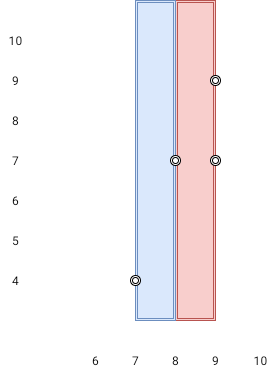1637. Widest Vertical Area Between Two Points Containing No Points

Given `n` `points` on a 2D plane where `points[i] = [xi, yi]`, Return the widest vertical area between two points such that no points are inside the area.

A vertical area is an area of fixed-width extending infinitely along the y-axis (i.e., infinite height). The widest vertical area is the one with the maximum width.

Note that points on the edge of a vertical area are not considered included in the area.

Example 1:```Input: points = [[8,7],[9,9],[7,4],[9,7]]
Output: 1
Explanation: Both the red and the blue area are optimal.
```

Example 2:

```Input: points = [[3,1],[9,0],[1,0],[1,4],[5,3],[8,8]]
Output: 3
```

Constraints:

• `n == points.length`
• `2 <= n <= 105`
• `points[i].length == 2`
• `0 <= xi, yi <= 109`

1637. Widest Vertical Area Between Two Points Containing No Points
``````struct Solution;

use std::collections::HashSet;

impl Solution {
fn max_width_of_vertical_area(points: Vec<Vec<i32>>) -> i32 {
let mut x_set: HashSet<i32> = HashSet::new();
for point in points {
x_set.insert(point);
}
let mut x_arr: Vec<i32> = x_set.into_iter().collect();
x_arr.sort_unstable();
let mut res = 0;
for w in x_arr.windows(2) {
res = res.max(w - w);
}
res
}
}

#[test]
fn test() {
let points = vec_vec_i32![[8, 7], [9, 9], [7, 4], [9, 7]];
let res = 1;
assert_eq!(Solution::max_width_of_vertical_area(points), res);
let points = vec_vec_i32![[3, 1], [9, 0], [1, 0], [1, 4], [5, 3], [8, 8]];
let res = 3;
assert_eq!(Solution::max_width_of_vertical_area(points), res);
}
``````